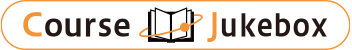### CourseDetailDegree
Bachelor
Course delivery methods
face-to-face
Subject
Mathematical sciences
Program
School
Institute of Mathematics ans Statistics (IME)
Department
Campus
São Paulo main campus
Classroom
Course Offering Year
Course Offering Month
January - January
Weekday and Period
Capacity
50
Credits
8
Language
English
Course Number
MAE5811

### Advanced Probability I University of Sao Paulo

#### Course Overview

The general treatment of Probability Theory requires its formulation in abstract spaces, in the framework introduced by Kolmogorov.

#### Learning Achievement

Introduce the basics of Probability Theory into abstract spaces, including the necessary elements of Measure Theory, in the framework formulated by Kolmogorov.

#### Course prerequisites

Exam and exercises, with the possibility of collecting an article at the end of the course.

#### Course schedule

1. Probability Spaces: (a) Lebesgue-Stieltjes Measure, Carath_dory Extension Theorem; (b) Measures of Probability, Random Variables; (c) Integration, Expectation, Convergence Theorems; (d) Product measures, Fubini's theorem; (e) Independence; (f) Kolmogorov Extension Theorem; (g) Radon-Nikodym Theorem, Conditional Expectation. 2. Laws of Large Numbers: (a) Convergence in Probability and Almost Sure Convergence; (b) Weak Law of Large Numbers; (c) Borel-Cantelli lemmas; (d) Strong Law of Large Numbers. 3. Central Limit Theorem: (a) Convergence in Distribution; (b) Characteristic Functions; (c) TLC for Random Variables I.I.D; (d) TLC for Triangular Arrangements.